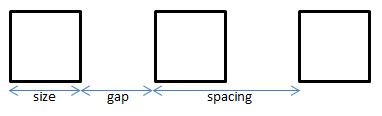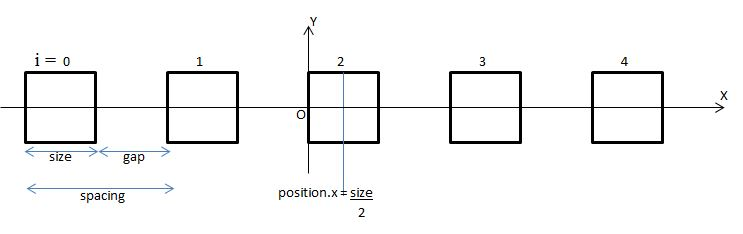# Vertical Wave with Vertex Shader

## Vertical Wave with Vertex Shader

As with any code it is important to have a firm idea of what you want to achieve, what is possible to code and a design.

## Requirements

The requirements are a row of many boxes that move vertically in a wave motion. The motion will be achieved by changing the the apparent positions of all vertices within a Vertex Shader. To be clear the positions of the boxes within the scene model will not be altered. What will be changed through the Vertex Shader is their projection onto the screen.

## First Stage Design

The boxes will be generated using the Solid Particle System as this deals with a multitude of repeated boxes efficiently. The vertical height of a box at any time will depend on the time and its position along the x axis using the sin function.

## First Challenge

Generally coding this project in Javascript, for example, would give access to the position of a box and so

box.position.y = Math.sin(box.position.x + time)

could be used.

However the data passed to a Vertex Shader Code is the attributes of the vertices of a mesh or is through using uniforms. Also the Vertex Shader Code applies to a single vertex with no access to any other vertices. Since the x coordinate of vertices on the left hand side of the box will differer by the size of the box to those on the right hand side. So sin(xLeft + time) with differ from sin(xRight + time) distorting the box.

What is needed is a method of obtaining the same number h, from the numbers xLeft and xRight.

## Second Stage Design

The boxes are cubes arranged equally spaced with the following parameters, size of box, gap between boxes and spacing = size + gapThe number of boxes n will be odd, numbered from the left starting with 0.

The box i will be at position (i - floor(n/2)) * spacing + size/2

For example when n = 5, floor(n/2) = 2 and the left hand edges of the boxes 0 to 4 will have positions

-2 * spacing, -1 * spacing, 0, 1 * spacing, 2 * spacing respectively. Adding size to these gives their hand edges.Dividing these left and right hand edge positions by spacing gives a pair of numbers for each box of

-2, -2 + (size/spacing),    -1, -1 + (size/spacing),    0, (size/spacing),    1, 1 + (size/spacing),    2, 2 + (size/spacing).

Since spacing = size + gap spacing > size and so (size/spacing) < 1 and it follows that applying the function floor to each of these numbers gives

-2, -2,    -1, -1,        0, 0,    1, 1,    2,2.

Hence obtaining a number h that is the same from the numbers xLeft and xRight.
For a box the x coordinate of any vertex will either be on a left hand or a right hand edge and so for each box x/spacing will give a unique number.

## Third Stage Design

Within the Vertex Shader Main function

vec3 p = position;float bn = floor(position.x / box_spacing);p.y = p.y + sin(time + bn/4.0);gl_Position = worldViewProjection * vec4(p, 1.0);

where time and box_spacing are uniforms.

## SPS Code

//Create SPS of Boxesvar boxes = 101; //odd numbervar box_size = 0.25; // must be floatvar box_gap = box_size/2;var box_spacing = box_size + box_gap;var box = BABYLON.MeshBuilder.CreateBox("box", {size:box_size}, scene);
var boxes_SPS = new BABYLON.SolidParticleSystem("boxesSPS", scene, {updatable: false});    //function to position boxesvar set_boxes = function(particle, i, s) {       var mid_point = Math.floor(boxes/2);    particle.position.x = (i - mid_point) * box_spacing + box_size/2;}
boxes_SPS.addShape(box, boxes, {positionFunction:set_boxes});  var boxes = boxes_SPS.buildMesh(); // mesh of leavesbox.dispose();

// Attributesattribute vec3 position;attribute vec3 normal;attribute vec2 uv;
// Uniformsuniform mat4 worldViewProjection;uniform float box_spacing;uniform float time;
// Normalvarying vec2 vUV;
void main(void) {    vec3 p = position;    float bn = floor(position.x / box_spacing);    p.y = p.y + sin(time + bn/4.0);    gl_Position = worldViewProjection * vec4(p, 1.0);
vUV = uv;}

varying vec2 vUV;
uniform sampler2D textureSampler;
void main(void) {  gl_FragColor = texture2D(textureSampler, vUV);}

### Set Material

var shaderMaterial = new BABYLON.ShaderMaterial("shader", scene, {		vertexElement: "vertexShaderCode",		fragmentElement: "fragmentShaderCode",	},	{		attributes: ["position", "normal", "uv"],		uniforms: ["world", "worldView", "worldViewProjection", "view", "projection"]	});

var mainTexture = new BABYLON.Texture("amiga.jpg", scene);
shaderMaterial.setTexture("textureSampler", mainTexture);

### Animation Loop

var time = 0;scene.registerBeforeRender(function() {    boxes.material.setFloat("time", time);    time +=0.1;});

BABYLONX.ShaderBuilder.InitializeEngine();		var shaderMaterial =   new BABYLONX.ShaderBuilder()             	.Solid({ b: 1 })	.SetUniform('box_spacing', 'float')    .Map({path:'amiga.jpg'  }) 	.VertexShader(        ' float bn = pos.x/box_spacing;\          result = vec4( pos.x, pos.y + sin(time + bn/4.0), pos.z  ,1.);')	.BuildMaterial(scene);

### Set Material

boxes.material = shaderMaterial;boxes.material.setFloat("box_spacing", box_spacing)

### Animation Loop

var time = 0;scene.registerBeforeRender(function () {
time += 0.1;
new BABYLONX.ShaderMaterialHelper().SetUniforms(        scene.meshes,        camera.position,        camera.target,        { x: 0, y: 0 },        { x: 100, y: 100 },        time);	            });